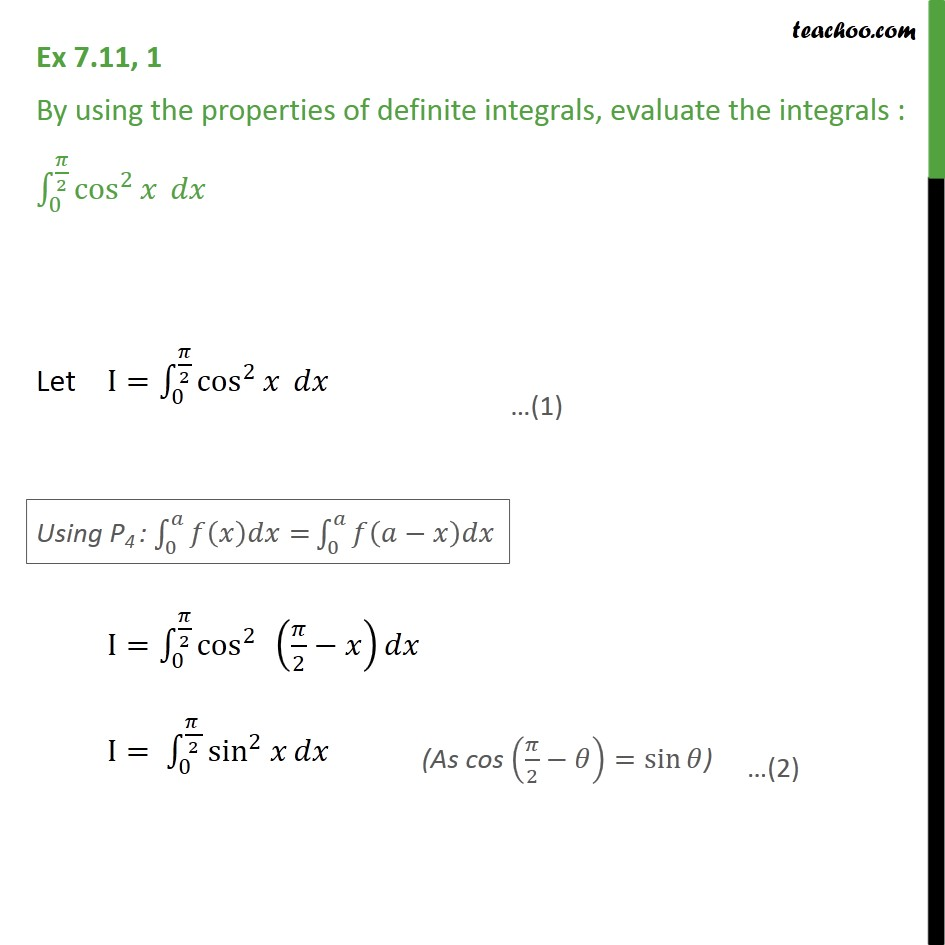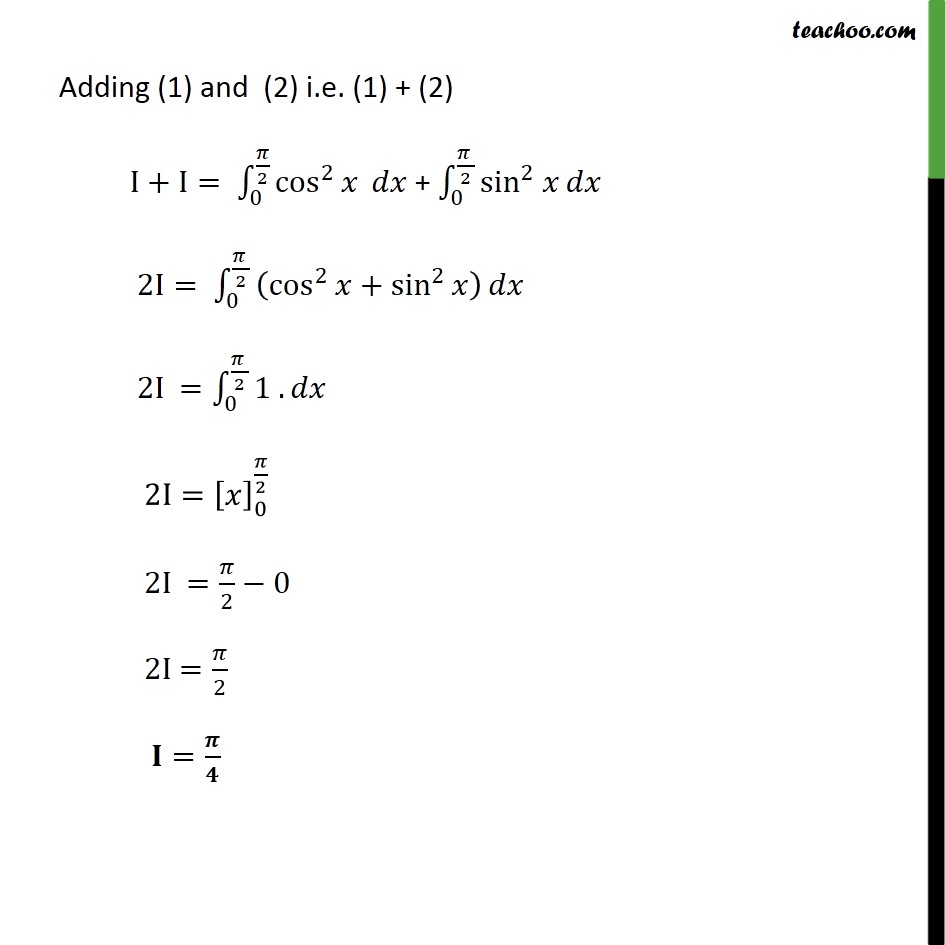1. Chapter 7 Class 12 Integrals (Term 2)
2. Serial order wise
3. Ex 7.11

Transcript

Ex 7.11, 1 By using the properties of definite integrals, evaluate the integrals : 0 2 cos 2 Let I= 0 2 cos 2 I= 0 2 cos 2 2 I= 0 2 sin 2 Adding (1) and (2) i.e. (1) + (2) I+I= 0 2 cos 2 + 0 2 sin 2 2I= 0 2 cos 2 + sin 2 2I = 0 2 1 . 2I= 0 2 2I = 2 0 2I = 2 =

Ex 7.11